Note: This is a working paper which will be expanded/updated frequently. All suggestions for improvement are welcome. The directory deleeuwpdx.net/pubfolders/mdsadd has a pdf version, the complete Rmd file with all code chunks, the bib file, and the R source code.

# 1 Introduction

We start with a ridiculously general MDS problem: each distance $$d_{ij}$$ is computed in its own configuration $$X_{ij}$$. It is possible that $$X_{ij}\not= X_{ji}$$, although we can suppose without loss of generality that $$X_{ii}=0$$ for all $$i$$. It is also possible that the $$X_{ij}$$ have different numbers of columns. Use $$\mathcal{X}$$ for the set of all $$X_{ij}$$.

Using standard notation we write $\begin{equation}\label{E:dist} d_{ij}^2(X_{ij}):=\mathbf{tr}\ X_{ij}'A_{ij}^{\ }X_{ij}^{\ }, \end{equation}$

with $$A_{ij}=(e_i-e_j)(e_i-e_j)'$$, where $$e_i$$ and $$e_j$$ are unit vectors (one element equal to one, the rest is zero). Not that we could also compute $$d_{k\ell}(X_{ij})$$ and $$d_{ij}(X_{kl})$$ but these cross-distances play no role in our techniques.

The stress loss function is $\begin{equation}\label{E:stress} \sigma(\mathcal{X}):=\sum_{i=1}^n\sum_{j=1}^n w_{ij}(\delta_{ij}-d_{ij}(X_{ij}))^2, \end{equation}$

where the $$w_{ij}$$ and $$\delta_{ij}$$ are non-negative weights and dissimilarities. We do not assume that $$W=\{w_{ij}\}$$ and $$\Delta=\{\delta_{ij}\}$$ are symmetric, but we assume without loss of generality that they are hollow, i.e. $$w_{ii}=\delta_{ii}=0$$ for all $$i$$. Minimizing stress over all possible $$\mathcal{X}$$ does not make sense, because we can always attain zero stress by choosing the $$X_{ij}$$ such that $$d_{ij}(X_{ij})=\delta_{ij}$$. Thus the problem only becomes interesting if there are restrictive constraints on the $$X_{ij}$$ that prohibit these trivial solutions.

## 1.1 smacof

Overviews of the smacof theory and algorithms for MDS are in De Leeuw (1977), De Leeuw and Heiser (1977), De Leeuw and Heiser (1980), Borg and Groenen (2005), De Leeuw and Mair (2009), Groenen and Van de Velden (2016). smacof stands for scaling by majorizing a convex function. We adapt the notation and results to our ridiculously general situation. For convenience assume that the dissimilarities are normalized so that the weighted sum of squares is equal to one. Expand stress as $\sigma(\mathcal{X})=1-2\sum_{i=1}^n\sum_{j=1}^n w_{ij}\delta_{ij}\ \sqrt{\mathbf{tr}\ X_{ij}'A_{ij}^{\ }X_{ij}^{\ }}+\sum_{i=1}^n\sum_{j=1}^n w_{ij}\ \mathbf{tr}\ X_{ij}'A_{ij}^{\ }X_{ij}^{\ }.$

We now apply majorization (a.k.a. MM) theory (De Leeuw (1994), Lange (2016)). Suppose we have two sets of configuration matrices $$\mathcal{X}$$ and $$\mathcal{Y}$$. Define the auxilary quantities $\xi_{ij}(X):=\begin{cases}\frac{\delta_{ij}}{d_{ij}(X)}&\text{ if }d_{ij}(X)>0,\\0&\text{ otherwise}.\end{cases}$ Note that $$d_{ij}(\xi_{ij}(X)X)=\delta_{ij}$$ for every $$X$$. Thus $$\xi_{ij}(X)$$ scales a configuration $$X$$ in such a way that the distance between points $$i$$ and $$j$$ is $$\delta_{ij}$$.

An application of Cauchy-Schwarz gives the majorization inequality $\begin{equation}\label{E:major} \sigma(\mathcal{X})\leq 1-2\sum_{i=1}^n\sum_{j=1}^nw_{ij}^{\ }\xi_{ij}(Y_{ij})\mathbf{tr}\ X_{ij}'A_{ij}^{\ }Y_{ij}^{\ }+\sum_{i=1}^n\sum_{j=1}^n w_{ij}^{\ }\mathbf{tr}\ X_{ij}'A_{ij}^{\ }X_{ij}^{\ }. \end{equation}$

The application of Cauchy-Schwarz to define the majorization function is what gives smacof its name. If we define $\rho(\mathcal{X},\mathcal{Y}):=\sum_{i=1}^n\sum_{j=1}^nw_{ij}\ \mathbf{tr}\ (X_{ij}-\xi_{ij}(Y_{ij})Y_{ij})'A_{ij}(X_{ij}-\xi_{ij}(Y_{ij})Y_{ij}),$

the majorization inequality $$\eqref{E:major}$$ can also be written as $$\sigma(\mathcal{X})\leq\rho(\mathcal{X},\mathcal{Y})$$ for all $$\mathcal{X}$$ and $$\mathcal{Y}$$, while $$\sigma(\mathcal{X})=\rho(\mathcal{X},\mathcal{X})$$ for all $$\mathcal{X}$$.

A smacof iteration in this general context is $\begin{equation}\label{E:smacof} \mathcal{X}^{(k+1)}=\mathop{\mathbf{argmin}}_{\mathcal{X}}\rho(\mathcal{X},\mathcal{X}^{(k)}) \end{equation}$

Of course there will be constraints on $$\mathcal{X}$$, otherwise the solutions is completely trivial and arbitrary.

## 1.2 Single Configuration Case

The minimization problem gets a more familiar look if we consider the case in which all $$X_{ij}$$ are constrained to be equal to a single configuration $$X$$. Then $X^{(k+1)}=\mathop{\mathbf{argmin}}_{X}\sum_{i=1}^n\sum_{j=1}^nw_{ij}\ \mathbf{tr}\ (X-\xi_{ij}(X^{(k)})X^{(k)})'A_{ij}(X-\xi_{ij}(X^{(k)})X^{(k)}),$ which has the solution, if there are no further constraints on $$X$$, $\begin{equation}\label{E:guttman} X^{(k+1)}=V^+B(X^{(k)})X^{(k)}, \end{equation}$ where $\begin{equation}\label{E:vmat} V:=\sum_{i=1}^n\sum_{j=1}^nw_{ij}\ A_{ij} \end{equation}$ and $\begin{equation}\label{E:bmat} B(X^{(k)}):=\sum_{i=1}^n\sum_{j=1}^nw_{ij}\xi_{ij}(^{(k)})A_{ij}. \end{equation}$

Equation $$\eqref{E:guttman}$$ defines what is known as the Guttman transform of $$X^{(k)}$$. The smacof algorithm iteratively applies Guttman transforms to the configuration matrix. De Leeuw (1988) shows linear convergence of these iterations, and De Leeuw (1984) shows that distances are non-zero at a local minimum.

We will first look at the special case in which the $$X_{ij}$$ can be partitioned into a common configuration and a parametric scalar component. Thus $$X_{ij}=\begin{bmatrix}X&\mid&\frac12\sqrt{2}f_{ij}(\theta)I\end{bmatrix}$$. The functions $$f_{ij}$$ are arbitrary, but we will discuss some interesting special cases.

As a consequence $$d_{ij}^2(X_{ij})=d_{ij}^2(X)+f_{ij}^2(\theta)$$. We have the partitioning $\rho(\mathcal{X},\mathcal{X}^{(k)})=\sum_{i=1}^n\sum_{j=1}^nw_{ij}\ \mathbf{tr}\ (X-\xi_{ij}(X_{ij}^{(k)})X^{(k)})'A_{ij}(X-\xi_{ij}(X_{ij}^{(k)})X^{(k)})+\sum_{i=1}^n\sum_{j=1}^nw_{ij} (f_{ij}(\theta)-\xi_{ij}(X_{ij}^{(k)})f_{ij}(\theta^{(k)}))^2,$ where $\xi(X_{ij}^{(k)})=\frac{\delta_{ij}}{\sqrt{d_{ij}^2(X^{(k)})+f_{ij}^2(\theta^{(k)})}}$ As a consequence a smacof step to update the configuration is simply $X^{(k+1)}=V^+B(X^{(k)})X^{(k)},$ with $B(X^{(k)})=\sum_{i=1}^n\sum_{j=1}^nw_{ij}\xi(X_{ij}^{(k)})A_{ij}.$ In the same way we update the additive part with $\xi^{(k+1)}=\mathop{\mathbf{argmin}}_\theta\sum_{i=1}^n\sum_{j=1}^nw_{ij}\ (f_{ij}(\theta)-\xi(X_{ij}^{(k)})f_{ij}(\theta^{(k)}))^2.$ Of course the neat part is that the two additive components can be optimized separately.

# 3 Applications

In the simplest case the additive components $$f_{ij}$$ are constants, not dependent on parameters that have to be estimated. This is analyzed in our first examples. We then analyze some more complicated cases, and even some cases in which we do not have additive components, although the general formulation still applies.

## 3.1 Regularized MDS

One problem with MDS is that there are configurations in which points coincide, and thus distances are zero. If $$w_{ij}\delta_{ij}>0$$ then De Leeuw (1984) shows that at a local minimum $$d_{ij}(X)>0$$. But this does not cover cases in which weights or dissimilarities are zero, or configurations which are not local minima. The problem can be avoided by choosing all $$f_{ij}$$ equal to a small $$\epsilon$$. The smacof iterations are Guttman transforms with $B(X^{(k)})=\sum_{i=1}^n\sum_{j=1}^nw_{ij}\ \frac{\delta_{ij}}{\sqrt{d_{ij}^2(X^{(k)})+\epsilon^2}}A_{ij}.$ It is interesting to look at the effect of this regularization on unidimensional scaling (De Leeuw (2005)). In unidimensional scaling we have $B(x^{(k)})x^{(k)}=\sum_{i=1}^n\sum_{j=1}^nw_{ij}\ \frac{\delta_{ij}}{|x_i^{(k)}-x_j^{(k)}|}(e_i-e_j)(x_i^{(k)}-x_j^{(k)})=\sum_{i=1}^n\sum_{j=1}^nw_{ij}\ \delta_{ij}\mathbf{sign}(x_i^{(k)}-x_j^{(k)})(e_i-e_j),$ which simplifies to $$B(x^{(k)})x^{(k)}=\tau^{(k)}$$, where $\tau_i^{(k)}=\sum_{j=1}^n (w_{ij}\delta_{ij}+w_{ji}\delta_{ji})\mathbf{sign}(x_i^{(k)}-x_j^{(k)}).$ Thus the update only depends on the rank order of the coordinates, and the algorithm converges in a finite number of iterations. This is no longer true if we regularize with a small $$\epsilon$$.

We illustrate with the Guilford vegetable data from the psych package (Revelle (2015)). These are paired comparison proportions $$p_{ij}$$, and according to Thurstone’s Case V model $$\Phi^{-1}(p_{ij})\approx x_i-x_j$$, which $$\Phi$$ the standard normal distribution function. Thus $$|\Phi^{-1}(p_{ij})|$$ should give us approximate unidimensional distances.

There are five different analyses, with five different values of $$\epsilon$$. The solutions, plotted in figure 1, as basically indistinguishable, until $$\epsilon$$ gets fairly large. The solution with $$\epsilon=0$$ is plotted as a red line. Increasing $$\epsilon$$ flattens the scale by drawing in the extremes, which is of course what regularization is supposed to do.

## f: 0.000  itel =    3 stress:    1.40614364
## f: 0.001  itel =    4 stress:    1.40613401
## f: 0.010  itel =    5 stress:    1.40518700
## f: 0.100  itel =    8 stress:    1.33982251
## f: 0.250  itel =   13 stress:    1.33907623
## f: 0.500  itel =   15 stress:    3.08078523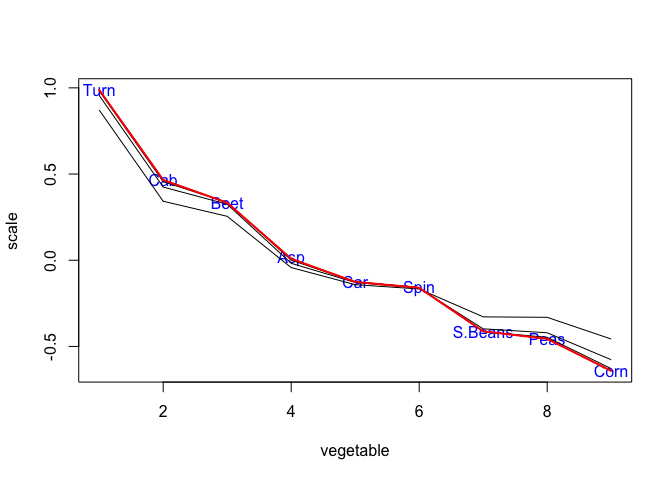Figure 1: Guilford Vegetable Data

## 3.2 Nested MDS

In principal component analysis and classical MDS solutions for different dimensionalities are nested, in the sense that the $$p$$-dimensional solution defines the first $$p$$ dimensions of the $$p+1$$-dimensional solution. One possible way around this problem is to define the $$p$$-dimensional solution as the first $$p$$ principal components of the full-dimensional MDS solution (De Leeuw, Groenen, and Mair (2016)). But we can also use additive components to go from the $$p$$-dimensional to the $$p+1$$-dimensional solution. Simply set the $$f_{ij}$$ equal to the distances of the $$p$$-dimensional configuration, and use $B(X^{(k)})=\sum_{i=1}^n\sum_{j=1}^nw_{ij}\ \xi_{ij}(X_{ij}^{(k)})A_{ij},$ with $\xi_{ij}(X_{ij}^{(k)})=\frac{\delta_{ij}}{\sqrt{(x_i^{(k)}-x_j^{(k)})^2+f_{ij}^2}}.$ to compute the next dimension.

As an illustration we use data from De Gruijter (1967), with average dissimilarity judgments between Dutch political parties in 1967.

##       KVP PvdA  VVD  ARP  CHU  CPN  PSP   BP
## PvdA 5.63
## VVD  5.27 6.72
## ARP  4.60 5.64 5.46
## CHU  4.80 6.22 4.97 3.20
## CPN  7.54 5.12 8.13 7.84 7.80
## PSP  6.73 4.59 7.55 6.73 7.08 4.08
## BP   7.18 7.22 6.90 7.28 6.96 6.34 6.88
## D66  6.17 5.47 4.67 6.13 6.04 7.42 6.36 7.36
The two-dimensional successive solution is in figure 2. It has stress 232.3973565646. The simultaneous two-dimensional solution is in figure 3, with stress 128.8832581227.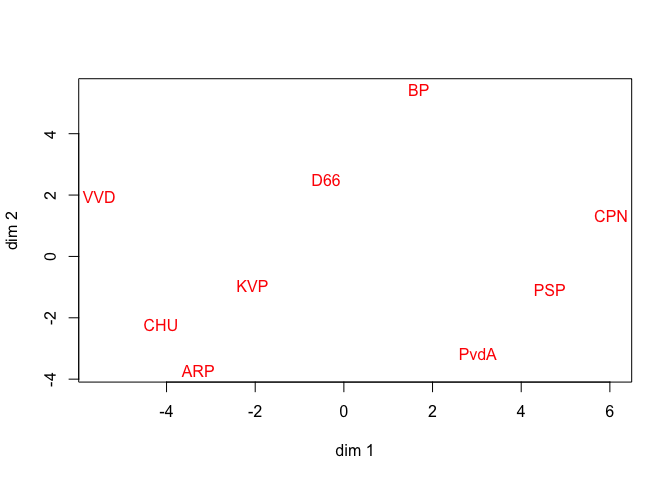Figure 2: De Gruijter Data, Successive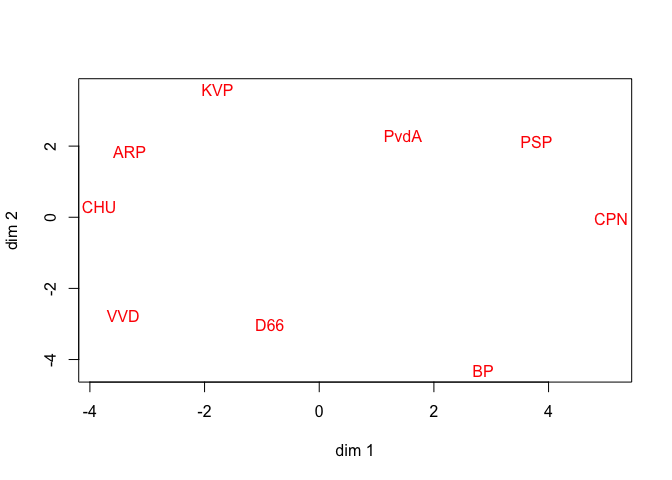Figure 3: De Gruijter Data, Simultaneous

In regularized MDS $$\epsilon$$ is a known constant. We can also design a version in which $$\epsilon$$ is estimated. The iteration for updating $$\epsilon$$ turns out to be $\epsilon^{(k+1)}=\epsilon^{(k)}\frac{\sum_{i=1}^n\sum_{j=1}^nw_{ij}\xi_{ij}(X_{ij}^{(k)})}{\sum_{i=1}^n\sum_{j=1}^nw_{ij}},$ with $\xi_{ij}(X_{ij}^{(k)})=\frac{\delta_{ij}}{\sqrt{d_{ij}^2(X^{(k)})+\{\epsilon^{(k)}\}^2}}.$ The De Gruijter data are suitable for illustrating the additive constant algorithm, because they are averaged dissimilarity judgments over a large number of students, and thus they are likely to exhibit regression to the mean.

The configuration

##              [,1]          [,2]
## KVP   1.795076832 -1.4883295209
## PvdA -1.449331623 -1.3662756501
## VVD   2.675413029  1.2333253971
## ARP   2.040024685 -1.3928429875
## CHU   2.317379999 -0.9477989531
## CPN  -3.992198052 -0.4130215421
## PSP  -2.954611015 -0.9587289598
## BP   -1.844521956  3.5848238952
## D66   1.412768101  1.7488483213
is given in figure 4. It requires 175 iterations and has stress 16.2605927675. Allowing estimation of an additive constant does not change the configuration very much, but stress is much lower than before. We do see the three Christian Democrat parties KVP, ARP, and CHU moving much closer together.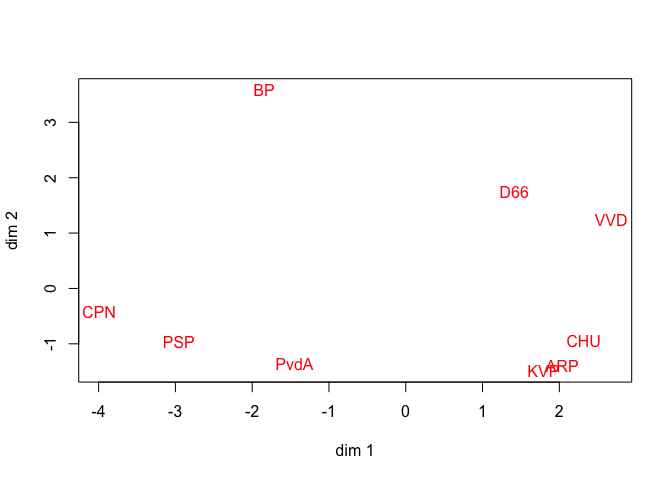Figure 4: De Gruijter Data, Additive Constant

## 3.4 MDS with Restrictions

Our examples so far can also be analyzed using the framework of De Leeuw and Heiser (1980). If all $$X_{ij}$$ are the same $\rho(X,X^{(k)})=\mathbf{tr}\ X'VX-2\mathbf{tr}\ X'B(X^{(k)})X^{(k)}+\mathbf{tr}\ (X^{(k)})'H(X^{(k)})X^{(k)},$ with $H(X^{(k)}):=\sum_{i=1}^n\sum_{j=1}^nw_{ij}\xi_{ij}^2(X^{(k)})A_{ij}.$ Thus a smacof step minimizes $\mathbf{tr}\ (X-V^+B(X^{(k)})X^{(k)})'V(X-V^+B(X^{(k)})X^{(k)}).$ Thus if there are restrictions on the possible configurations, say $$X\in\Omega$$, then we first compute the Guttman transform and then its (weighted) least squares projection on the constraint set $$\Omega$$.

For all problems in which the common configuration is partitioned into two parts $$\begin{bmatrix}X_1&\mid&X_2\end{bmatrix}$$ we have separation of the loss components \begin{align*} \rho(X,X^{(k)})&=\mathbf{tr}\ (X_1-V^+B(X^{(k)})X^{(k)}_1)'V(X_1-V^+B(X^{(k)})X^{(k)}_1)\\&+\mathbf{tr}\ (X_2-V^+B(X^{(k)})X^{(k)}_2)'V(X_2-V^+B(X^{(k)})X^{(k)}_2). \end{align*}

For the regularized MDS and additive constant problems $$X$$ is of the form $$\begin{bmatrix}X_1&\mid&\epsilon I\end{bmatrix}$$, for nested MDS we have $$\begin{bmatrix}X_1&\mid&X_2\end{bmatrix}$$, with $$X_1$$ the fixed lower-dimensional solution. Thus for the regularized MDS problem and for the additive constant problem $$X^{(k+1)}_1=V^+B(X^{(k)})X^{(k)}_1$$, and for the additiive constant problem $\epsilon^{(k+1)}=\frac{\mathbf{tr}\ VV^+B(X^{(k)})X^{(k)}_2}{\mathbf{tr}\ V}=\epsilon^{(k)}\frac{\mathbf{tr}\ B(X^{(k)})}{\mathbf{tr}\ V}.$ For nested MDS simply $$X_2^{(k+1)}=V^+B(X^{(k)})X^{(k)}_2$$.

# 4 Partitioned Dissimilarities

## 4.1 Piecewise MDS

Consider the following situation. The dissimilarities are partitioned into, say, $$m$$ groups, and the configurations $$X_\ell$$ are constrained as $$X_\ell=X\Lambda_\ell$$ with $$\Lambda_\ell$$ diagonal. The groups of dissimilarities are arbitrary. Perhaps dissimilarities come from different sources, or have different degrees of precision.

We shall treat the general case in which both $$X$$ and the $$\Lambda_\ell$$ must be estimated. The case in which the $$\Lambda_\ell$$ are fixed and known is included as a special case in the general treatment. In iteration $$k$$ we must minimize $\begin{equation}\label{E:ploss} \sum_{\ell=1}^m\sum_{(i,j)\in\mathcal{I}_\ell}w_{ij}\ \mathbf{tr}\ (X\Lambda_\ell-\xi_{ij}(X_\ell^{(k)})X_\ell^{k})'A_{ij}(X\Lambda_\ell-\xi_{ij}(X_\ell^{(k)})X_\ell^{k}), \end{equation}$ which becomes $\sum_{\ell=1}^m\mathbf{tr}\ \Lambda_\ell X'V_\ell X\Lambda_\ell-2\sum_{\ell=1}^m\mathbf{tr}\ \Lambda_\ell X'B_\ell(X_\ell^{(k)})X_\ell^{(k)}+\sum_{\ell=1}^m\mathbf{tr}\ (X_l^{(k)})'H_\ell X_l^{(k)}.$ The stationary equations are $\begin{equation}\label{E:stat1} \sum_{\ell=1}^m V_\ell X\Lambda_\ell^2=\sum_{\ell=1}^mB_\ell(X_\ell^{(k)})X_\ell^{(k)}\Lambda_\ell \end{equation}$ and, in the case we are also minimizing over $$\Lambda_\ell$$, $\begin{equation}\label{E:stat2} \Lambda_\ell=\frac{\mathbf{diag}(X'B_\ell(X_\ell^{(k)})X_\ell^{(k)})}{\mathbf{diag}(X'V_\ell X)} \end{equation}$

There is little hope to solve these two equations analytically, so we resort to alternating least squares. It is easy enough to solve $$\eqref{E:stat2}$$ for the $$\Lambda_\ell$$ for given $$X$$. To solve $$\eqref{E:stat1}$$ for $$X$$ for given $$\Lambda_\ell$$ consider column $$s$$ from both sides of the equation. This gives $x_s=\left[\sum_{\ell=1}^m\Lambda_{ls}^2V_\ell^{\ }\right]^+u_s,$ where $$u_s$$ is column $$s$$ of the matrix on the right-hand side of $$\eqref{E:stat1}$$. Note that if at some point a diagonal element of $$\Lambda_\ell$$ is zero, it will remain zero in subsequent iterations. Thus we can impose zeroes in the $$\Lambda_\ell$$ by fiLambdang them in the initial estimate.

The De Gruijter data show the familar bending or horseshoe effect, where the left right scale is bended to bring the extreme left and right closer than they would otherwise be. We first analyze the data with the psmacof function, using a single common dimension and with another single dimension for the largest dissimilarities. The cutoff is the median of the $$\delta_{ij}$$, which is 6.35. Thus there are two groups: for the smaller dissimilarities below the median both only the first dimension gets unit weight, for the larger dissimilarities both dimensions have unit weight. The $$\Lambda_\ell$$ are fixed, and there is no need to minimize over them.

After 143 iterations we convergence on the configuration

##               [,1]          [,2]
## KVP   1.0813664617 -2.2452423226
## PvdA -2.5518200495 -1.2956894362
## VVD   4.0227369242 -0.2114471130
## ARP   2.6197105314 -1.3322490334
## CHU   4.9836790756  0.5617603512
## CPN  -5.6188008950  1.2897569555
## PSP  -3.8704005788  1.4107253694
## BP    0.5384787791  5.2408076168
## D66  -1.2049502488 -3.4184223877
which is plotted in figure 5 and has stress 267.5183648725.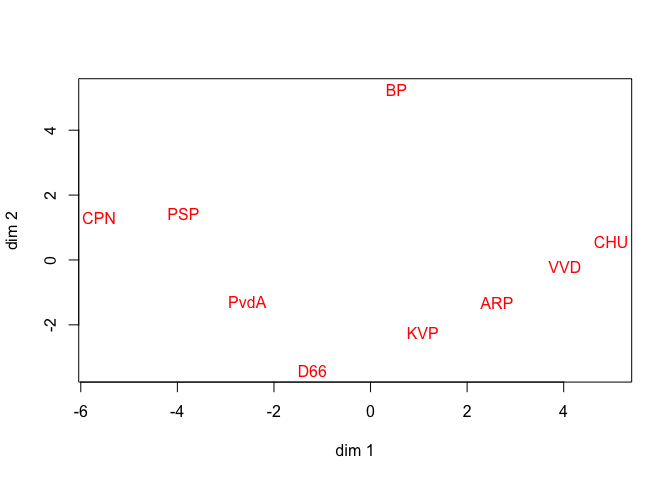Figure 5: De Gruijter Data, Piecewise 1 + 1

In the next psmacof analysis, of the same data, we have two common dimensions and one extra dimension for the large dissimilarities. We also allow optimization over the $$\Lambda_\ell$$.

The configuration is

##               [,1]          [,2]          [,3]
## KVP   0.7186122805 -3.7503570828  3.8641669251
## PvdA -1.8106871731 -2.1504563824 -0.4253678930
## VVD   2.0428676791  2.3164244036 -3.8180734743
## ARP   2.5691368914 -2.8077972844 -0.2075449599
## CHU   3.1007780793 -0.9687224372 -1.9774708918
## CPN  -2.8390433190  2.5465243233  3.0219947275
## PSP  -4.7186655261 -0.3376897597  0.5033057048
## BP    1.4759117466  3.1106775204  3.1092399708
## D66  -0.5389106586  2.0413966992 -4.0702501092
and we plot it in figure 6. It requires 1816 iterations and has stress 27.684120348.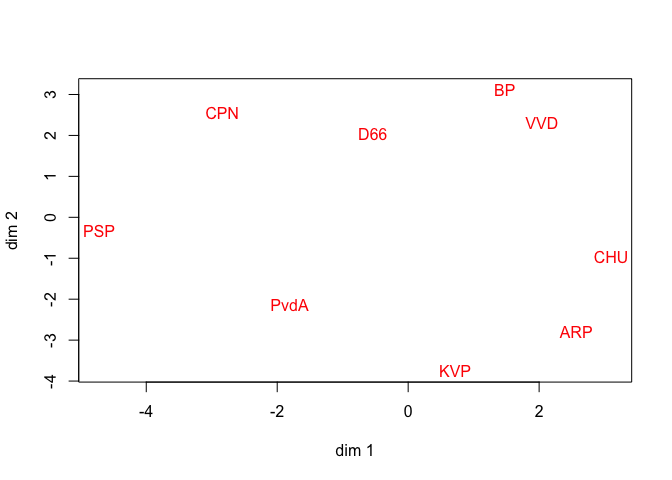Figure 6: De Gruijter Data, Piecewise 2 + 1

The algorithm becomes only slightly more complicated if we do not require the $$\Lambda_\ell$$ to be diagonal, but allow them to be full matrices.

## 4.2 INDSCAL/PARAFAC

An interesting special case of $$\eqref{E:ploss}$$, where the dissimilarity groups are defined by different individuals or occasions or time-points, is $\sum_{\ell=1}^m\sum_{i=1}^n\sum_{j=1}^n w_{ij\ell}\ \mathbf{tr}\ (X\Lambda_\ell-\xi_{ij\ell}(X_\ell^{(k)})X_\ell^{k})'A_{ij}(X\Lambda_\ell-\xi_{ij\ell}(X_\ell^{(k)})X_\ell^{k}),$ with $\xi_{ij\ell}(X_\ell)=\frac{\delta_{ij\ell}}{d_{ij}(X_\ell)}$

Now piecewise MDS with $$m$$ groups is the smacof algorithm for INDSCAL, in which we optimize over the $$\Lambda_\ell$$ and use the same common configuration for each group. Again, this is easily extended to matrices $$\Lambda_\ell$$ which are not restricted to be diagonal, thus giving the smacof algorithm for IDIOSCAL.

We use the color data of Helm (1959) to illustate the ismacof function. There are 12 individuals, the first ten with normal color vision (N), the last two with color deficiency (CD). For individual N6 and individual CD2 the experiment was replicated.

After 494 iterations we find stress 2542.2372780397. In figure 7 we give the common configuration $$X$$, and in figure 12. Note in figure 12 the color deficient subjects are in blue, the color normal subjects are in red. In a real analysis of these data we would probably conclude that two dimensions was not enough to show important variation. The stress measures for each of the 16 individuals, including two replications, are

##     N1     N2     N3     N4     N5    N6a    N6b     N7     N8     N9
##  57.26 208.61  88.45  76.56 111.57  94.32  48.39 116.07 148.45 125.86
##    N10    CD1   CD2a   CD2b    CD3    CD4
## 162.94 202.58 279.83 240.64 387.40 193.32
and thus stress is much larger for color deficient individuals.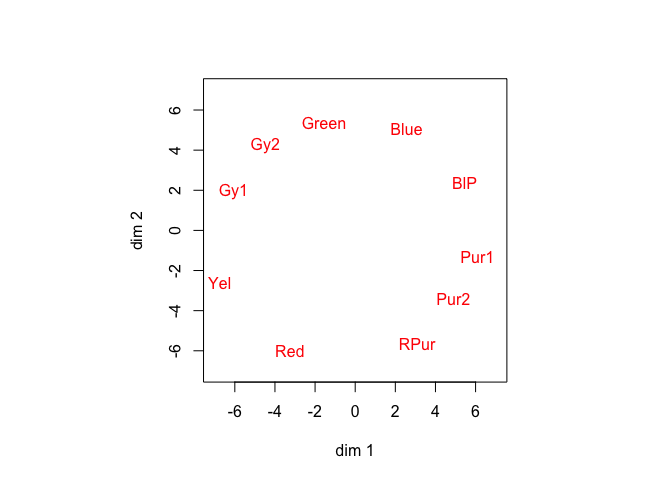Figure 7: Helm Data, Configuration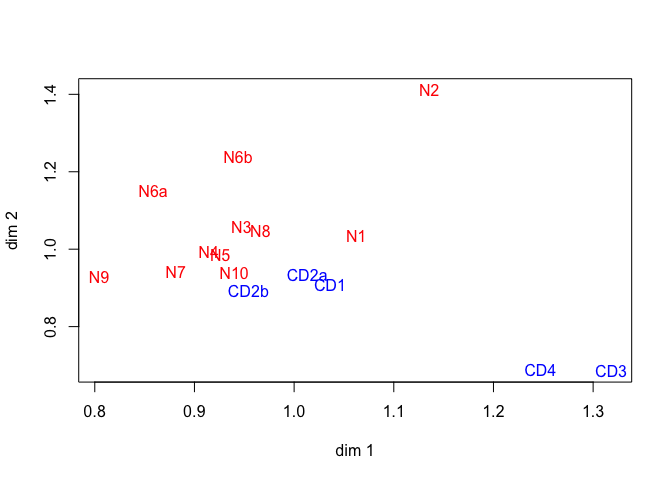Figure 8: Helm Data, Subject Weights

# 5 Modeling Asymmetry

## 5.1 Slide-vector

The slide vector model for asymmetric dissimilarities was proposed, but never published, by Kruskal around 1973. It was discussed in detail by Zielman and Heiser (1993). It assumes, in our notation, that $$X_{ij} = X + \frac12(e_i-e_j)z'$$ and $$X_{ji} = X + \frac12(e_j-e_i)z' = X - \frac12(e_i-e_j)z'$$, so that $$\frac12(X_{ij}+X_{ji})=X$$ and $$(X_{ij}-X_{ji})=(e_i-e_j)z'$$. In terms of the squared distances $d_{ij}^2(X_{ij})=\mathbf{tr}\ X_{ij}'A_{ij}^{\ }X_{ij}^{\ }=(x_i-x_j+z)'(x_i-x_j+z).$

Define $T:=\begin{bmatrix}X\\z\end{bmatrix}$ and $$u_{ij}$$ is a vector of length $$n+1$$ with zeroes, except for elements $$i$$ and $$n+1$$, which are $$+1$$, and element $$j$$, which is $$-1$$. Thus $$T'u_{ij}=(x_i-x_j+z)$$ and $$d_{ij}^2(X_{ij})=u_{ij}'TT'u_{ij}$$.

It follows that a smacof step is $\begin{equation}\label{E:slide} T^{(k+1)}=\left\{\sum_{i=1}^n\sum_{j=1}^nw_{ij}u_{ij}u_{ij}'\right\}^+\left\{\sum_{i=1}^n\sum_{j=1}^nw_{ij}\xi_{ij}(X_{ij}^{(k)})u_{ij}u_{ij}'\right\}T^{(k)}, \end{equation}$

with $\xi_{ij}(X_{ij}^{(k)})=\frac{\delta_{ij}}{d_{ij}(X_{ij}^{(k)})}.$ This can be clarified somewhat, by using $u_{ij}u_{ij}'=\begin{bmatrix}A_{ij}&(e_i-e_j)\$$e_i-e_j)'&1\end{bmatrix},$ so that, for example, $\sum_{i=1}^n\sum_{j=1}^nw_{ij}u_{ij}u_{ij}'=\begin{bmatrix}V&\sum_{i=1}^n\sum_{j=1}^n w_{ij}(e_i-e_j)\\\sum_{i=1}^n\sum_{j=1}^nw_{ij}(e_i-e_j)'&\sum_{i=1}^n\sum_{j=1}^n w_{ij}\end{bmatrix}.$ Note that if \(W=\{w_{ij}\}$$ is symmetric, then $$\sum_{i=1}^n\sum_{j=1}^nw_{ij}(e_i-e_j)=0$$, which simplifies the matrix generalized inverse in $$\eqref{E:slide}$$.

We apply the function ssmacof to the brand switching of nine green and seven blended bottled teas given by Taniola and Yadohisa (2016). The frequencies of switching $$n_{ij}$$ are transformed to asymmetric dissimilarities with $$\delta_{ij}=\sqrt{n_{ii}+n_{jj}-2n_{ij}}$$.

##      DG  IG1 IG2 IG3 KaG SaG SG1 SG2 7G  ABl CBl1 CBl2 CBl3 KaBl KBl1 KBl2
## DG    32   0   0   0   0   0   0   0   0   0   0    0    0    0    1    0
## IG1    2 283   0  18   2   2   1  40   0   0   0    5   18    0   17    2
## IG2    2  41 177   9   0   2   1  13   0   0   0    2   10    0    6    0
## IG3    0   0   0  18   2   0   3   9   0   0   1    2    8    0    9    0
## KaG    0   0   0   0  58   0   1   3   0   0   0    1    1    6    0    1
## SaG    2   0   0   0   0  24   0   0   0   0   0    0    0    0    2    0
## SG1    0   0   0   0   0   1  14   0   0   0   0    1    2    0    8    0
## SG2    3   0   0   0   0   3   8 246   0   0   2    2   26    0   21    1
## 7G     0   4   5   0   0   0   0   3  56   0   0    1    1    0    2    0
## ABl    0  15  11   5   0   0   2  11   4 137   2    5   16    2    5    2
## CBl1   0   0   0   0   0   0   0   0   0   0  22    0    0    0    3    1
## CBl2   0   0   0   0   0   3   0   0   0   0   4  114    0    0   13    7
## CBl3   2   0   0   0   0   5   0   0   0   0   7   22  201    0   23    5
## KaBl   0   0   0   0   0   0   0   2   0   0   0    3    2   28    1    2
## KBl1   0   0   0   0   0   0   0   0   0   0   0    0    0    0  192   13
## KBl2   0   0   0   0   0   0   0   0   0   0   0    0    0    0    0    5

The slide vector algorithm takes 1199 iterations to find a solution with stress 2844.4928948188. The slide vector $$z$$ is , and the configuration

##      [,1]     [,2]
## DG   +1.6003  +3.5210
## IG1  +14.8848 +6.2248
## IG2  +10.5817 -7.4227
## IG3  +1.7787  -1.3772
## KaG  -5.6537  +1.7343
## SaG  -1.3980  +2.4636
## SG1  +0.4404  +0.4537
## SG2  +2.1264  +14.7160
## 7G   +5.6544  +0.1990
## ABl  +1.7900  -11.4184
## CBl1 -2.6166  -1.9803
## CBl2 -5.6584  -8.6451
## CBl3 -9.7128  +9.4961
## KaBl +0.2821  -3.9134
## KBl1 -13.3137 -3.2594
## KBl2 -0.7857  -0.7921
is plotted in figure 9 (green teas in blue, blended teas in red).